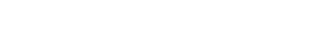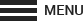# Life-Cycle Cost Analysis: Step 7 - Compute Life-Cycle Costs

Using the parameters presented in this training calculate the present worth of each alternative.

## HISTORIC APPROACH

1. Calculate the cost to develop and construct the alternative.
2. Add the present worth of future repair costs.
3. Subtract the present worth of the service life of the initial construction and subsequent repairs remaining at the end of the analysis period.

Calculate the life cycle cost and Benefit/Cost Ratio using the Historic Approach:

Worksheet: Excel | PDF
Instructions: PDF

## HEC-17 APPROACH

1. Calculate the annual risk of future repair costs by multiplying the difference in exceedance probability by the average repair cost for each pair of events (10yr-25yr, 25yr-50yr, etc.)
2. Sum the annual risks and calculate the present worth of the annual series over the analysis period.
3. Add the cost to develop and construct the alternative; adjusted by the service life remaining at the end of the analysis period.

Calculate the life cycle cost and Benefit/Cost Ratio using the HEC-17 Approach:

Worksheet: Excel | PDF
Instructions: PDF

## HISTORIC AND HEC-17 APPROACH

Download the spreadsheets for calculating the life cycle cost and Benefit/Cost Ratio using the Historic and HEC-17 Approaches. Supporting documentation must be provided for each variable input into the spreadsheet. Cost estimates must show quantities of major construction items such as earthwork, surfacing, and wall area. Costs input into the spreadsheets must be the Real Cost (the cost of the repair today.)

The Benefit/Cost Ratio is calculated as follows:

• The Benefit is the reduction of future repair costs (adjusted by the remaining service life of previous repairs when using the Historic Approach).
• The Cost is the difference between the construction costs of the two alternatives (adjusted by the remaining service life of the initial investment).